Editor Ratings:
User Ratings:
[Total: 0 Average: 0]

Here’s a list of 10 websites to calculate inverse of matrix within just few steps. The Inverse is one of the important topics of whole Matrix concept in Mathematics. There are different methods for calculating the inverse of the matrix which you can follow. The thing is that not every square matrix has an inverse so it can be a little annoying to check each and every matrix to check which one has an inverse and which one not.

So you can simply use any of these websites to calculate inverse of matrix within seconds and save a lot of time. So let’s get started with them.

## 1. Bluebit: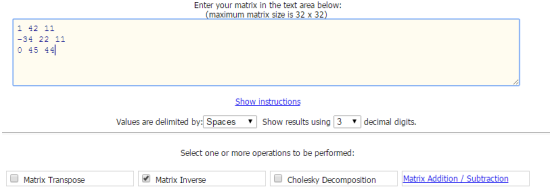Bluebit is the first inverse calculator for matrix in this list. You can use Bluebit to calculate inverse of matrix of order up to 32. To enter the values, you have to use keyboard to input values and you can use comma, space, or tabs as the separator. The Bluebit also lets you choose the number of decimal digits you want to use.

The website displays result on the next page along with the matrix entered by you. Besides calculating inverse, you can also use it to calculate transpose, trace, determinant, rank, decomposition, etc..

## 2. Math Portal: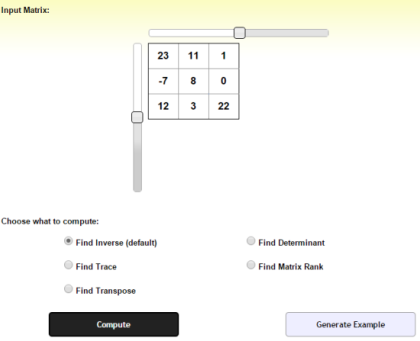Math Portal is the next website to calculate inverse of the matrix. It has sliders for setting the order of the matrix which you want to solve. The website supports matrices of order up to 6 and you have to enter the values in the cell. When you have clicked on the Compute option, you will see the result along with the input matrix. Apart from this, Math Portal also lets you calculate trace, transpose, determinant, and matrix rank.

## 3. Math Is Fun:Math Is Fun is the third inverse calculator for you to try it out and calculate inverse of matrices. This website lets you solve the inverse of matrices of order up to 10. It includes individual cells for you to enter the values and sliders for choosing the order of matrix. When you have entered the matrix, click on inv(A) to get the solution.

You can also use Math is Fun to solve addition, subtraction, determinant, multiplication, and many other problems related to matrices.

## 4. Number Empire:The fourth inverse calculator is Number Empire in this list. The Number Empire supports matrices of order up to 40 and you can use to solve any problem of matrix as long as the order is 40 or less. You have to enter the values by separating them with spaces.

The Number Empire can also be used for solving determinant, transpose, rank, triangular matrix, etc..

## 5. Matrix Calculator: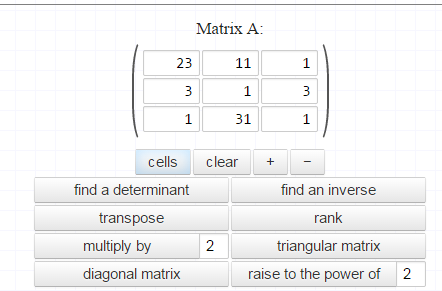Matrix Calculator is an inverse calculator which doesn’t mention the maximum supported matrix order for solving the problem. It has + and – marked buttons which you can click to increase and decrease the order respectively. You have to enter the values of the problem and then click on Find an Inverse to get the answer.

Like all other inverse calculator mentioned above, this one also lets you calculate a lot of other problems like transpose, diagonal matrix, raise the power, triangular matrix etc..

## 6. Solve My Math: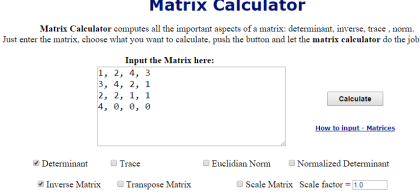The sixth web site to calculate inverse of a matrix is Solve My Math. It doesn’t come with individually marked cells for entering the values and you have to enter them by separating using commas. On entering the values, you have to check the option Inverse Matrix and you will get the answer.

The other problems which you can solve using this website are determinant, trace, transpose, euclidian, etc..

## 7. Matrix Reshish: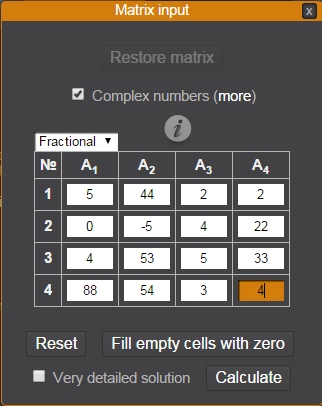Matrix Reshish is the inverse calculator which supports maximum number of order which goes up to 100. Simply choose the order from menu, enter values and click on Calculate to get the answers. It also gives you maximum choices while solving the problem. You can choose whether you want answers in fraction or decimal, option to auto fill zeroes in all blank cells, and option for getting detailed solution.

## 8. QuickMath:QuickMath is the simplest inverse calculator in this list. You simply have to enter the values and click on Inverse option to get the solution. The QuickMath doesn’t offer any options for you to change. You have to use comma as the separator for differentiating between individual values.

You can also go for checking step by step solution when you have got the option. The QuickMath doesn’t show them by default.

## 9. OnlineMSchool: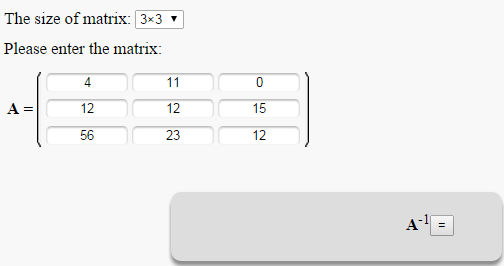The second last inverse calculator in this list is OnlineMSchool. It lets you solve the matrices of order up to 7. The steps to solve the matrix are simple like others above. You simply need to enter the values in the individual cells and click on the Inverse button to get the solution.

The good thing is that it displays complete solution step by step which helps in better understanding and grasp over the concept of inverse.

## 10. TutorVista: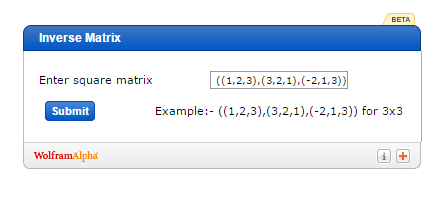The final inverse calculator in the list is TutorVista. It supports solving matrix problems up to order 4. However, this calculator differs in the way of entering the values of the problem for solving. You have to enter the problem in following order: ((a,b,c), (d,e,f), (g,h,i)) for solving a matrix of order 3. Just enter the problem in this order and click on Submit to view the answer.

Update 2022: TutorVista doesn’t exist anymore. We recommend you use Wolfram Alpha instead.

These were the 10 websites to calculate inverse of any matrix. Do give them a try and check out which one works for you best. For any problems or doubts, you can get in touch with me using the comments section below.

You may also like: 10 Websites to Calculate Determinant of a Matrix.

 Editor Ratings: User Ratings:[Total: 0 Average: 0] Free/Paid: Free